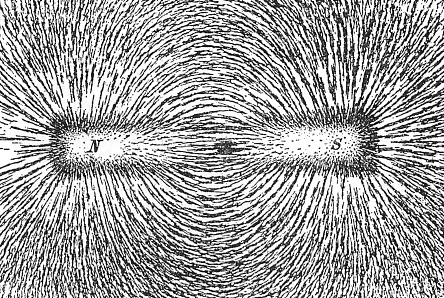Amagnetic fieldFigure 1. Magnetic field around a bar magnet.

A magnetic field is a field of force that is generated by a magnet and a conductor through which an electric current is flowing; or, equivalently, a region in which magnetic forces can be observed.

Magnetic poles

Magnetic poles are the field region in which is magnetism appears to be concentrated. If a bar magnet is suspended to swing freely in the horizontal plane, one pole will point north; this is called the north-seeking or north pole. The other pole, the south-seeking or south pole, will point south. Unlike poles attract each other; like poles repel. Earth's magnetic poles are the result of the huge "magnet" that is the Earth.

Magnetic field lines

A magnetic field can be represented by a set of lines of force (flux lines) spreading out from the poles of a magnet or running around a current-carrying conductor. These lines of forces can be seen if iron filings are sprinkled on to a sheet of paper below which a magnet is placed (see Figure 1). The filings line up along the lines of force, the density of the lines being greatest where the field is strongest. At any point in space, the local field line points in the direction of the magnetic force which an isolated magnetic pole at that point would experience.

Magnetic field strength

Within a magnetic field a magnetic dipole may experience a torque and a moving charge may experience a force. A magnetic field with its associated electric field is called an electromagnetic field.

The magnetic field strength (H) is one of two quantities that specifies both the strength and direction of a magnetic field; the other is magnetic flux density (B). The magnetic field strength (H) is related to B by: H = B/μ, where μ is the permeability of the medium. The SI unit of field strength is the ampere per meter (A m-1). Magnetic field strength is a vector quantity.